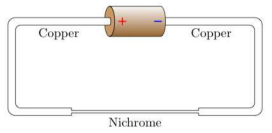# Problem: In the circuit shown, two thick copper wires connect a 1.5 V battery to a Nichrome wire. Each copper wire has radius R = 8 mm and is L = 17 cm long. Copper has 8.4 × 10 28 mobile electrons per cubic meter and an electron mobility of 4.4×10 −3 (m/s)/(V/m). The Nichrome wire is l = 7 cm long and has radius r = 3 mm. Nichrome has 9 × 1028 mobile electrons/m3 and an electron mobility of 7 × 10−5 (m/s)/(V/m). What is the magnitude of the electric field in the copper wire? 1. 0.00315418 2. 0.0507735 3. 0.085777 4. 0.0399455 5. 0.00295718 6. 0.0775274 7. 0.0210359 8. 0.0670841 9. 0.0560538 10. 0.103878

🤓 Based on our data, we think this question is relevant for Professor Geier's class at CSULB.

###### Problem Details

In the circuit shown, two thick copper wires connect a 1.5 V battery to a Nichrome wire. Each copper wire has radius R = 8 mm and is L = 17 cm long. Copper has 8.4 × 10 28 mobile electrons per cubic meter and an electron mobility of 4.4×10 −3 (m/s)/(V/m). The Nichrome wire is l = 7 cm long and has radius r = 3 mm. Nichrome has 9 × 1028 mobile electrons/mand an electron mobility of 7 × 10−5 (m/s)/(V/m). What is the magnitude of the electric field in the copper wire?

1. 0.00315418

2. 0.0507735

3. 0.085777

4. 0.0399455

5. 0.00295718

6. 0.0775274

7. 0.0210359

8. 0.0670841

9. 0.0560538

10. 0.103878